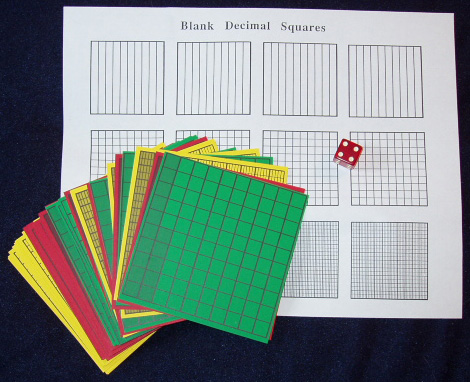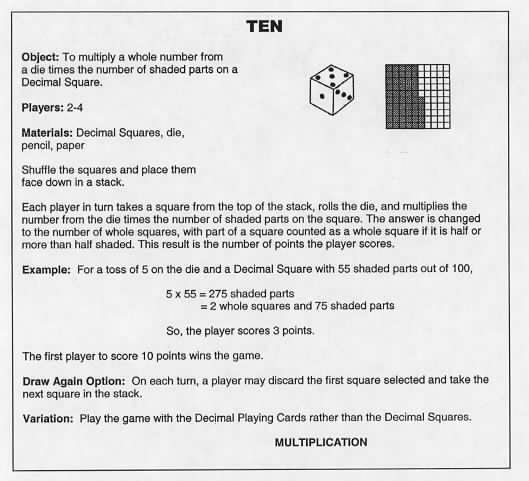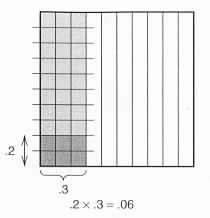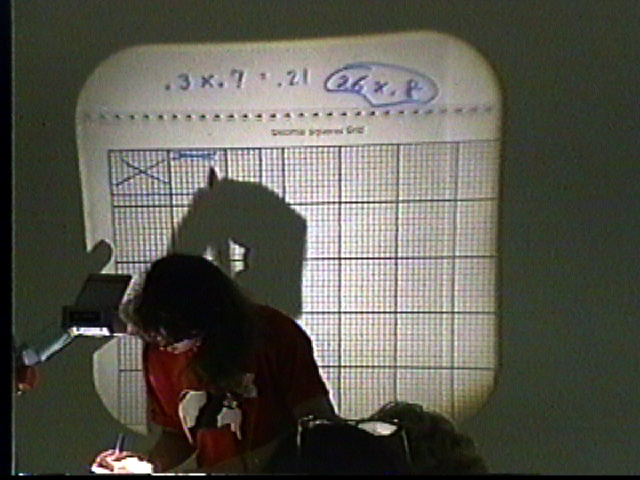Home
 Multiplication . Materials: Deck of Decimal Squares and one die per group and sheets of Blank Decimal Squares for Tenths Hundredths and Thousandths .Multiplying a Whole Number Times a Decimal Play the game TEN* if time permits. (see directions below) Place an arbitrary transparent square on the overhead and roll a die. Multiply the number from the die times the number of shaded parts and note that the score for the turn is the total shaded amount rounded off to the nearest whole square. For the example in the game directions below the player scores 3 points. Explain the game of TEN and the Draw Again Option. Teachers will play this game in pairs and each pair will need a die. Calculators may be used for whole number computations. This game is also on page 90 of the Decimal Squares Teacher’s Guide.Following the game discuss the strategies used. In particular ask for the least and greatest possible scores for a given turn. Discuss how this game provides readiness for multiplication of a whole number times a decimal. For example for a green square with 35 parts shaded and a roll of 5 we compute 5 x 35 = 175 and then convert this to one whole square and 75 parts out of 100. We do the same computation when multiplying 5 times the decimal .35; that is we multiply 5 times the whole number 35 and then locate the decimal point to obtain 1.75. . Activity - Shade Blank Decimal Squares to illustrate the following operations: 3 x .6; 4 x .42; and 3 x .395. The students in this photo are playing the game Ten. One of the products on the paper for the student in the foreground shows 5 x 35 (35 parts out of 100) and beneath is the product 175. Rounded to the nearest whole square this results in a score of 2 points.Photo courtesy of Herb Moyer Multiplying a Decimal Times a Decimal Place the transparency of decimal relations and operations below on the overhead and ask each teacher to select one or two and use blank Decimal Squares to explain why they are true. Ask for demonstrations of a few at the overhead. Some teachers may need help with a visual demonstration of a decimal times a decimal. For example x .3 means to take 2-tenths of 3-tenths which is shown at the right by dividing the shaded amount of a .3 square into 10 equal parts. This can also be illustrated with transparent squares by placing the square for .2 on top of the square for .3 and at right angles to it. The two sets of lines make 100 small parts and the intersection of the shaded regions is .06 of a whole square.Activity - Shade Blank Decimal Squares to illustrate the following operations: .3 x .6; .4 x .3; and .2 x .7. Multiplication involving decimals and mixed decimals can also be modeled by grids as is being shown by the following student at the overhead.